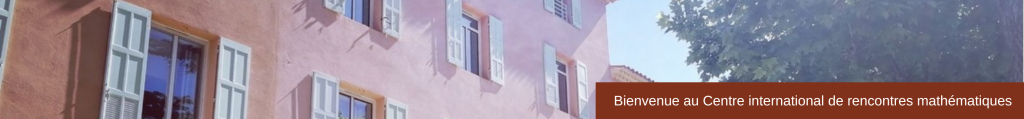## Recent Trends in Nonlinear Evolution EquationsApril 4 – 8, 2016

Dispersive estimates for the Schrödinger equation on 2-step stratified Lie groups  (pdf)

Concentration sets for multiple equal depth wells potentials in the 2D elliptic case  (pdf)

Birkhoff normal form for null form wave equations  (pdf)

Ancient solutions to geometric flows  (pdf)

Blow-up by bubbling in some critical parabolic equations  (pdf)

Transition Fronts for Monostable Reaction-Diffusion Equations  (pdf)

Propagation of regularity of solutions to the k-generalized Korteweg-de Vries equation (pdf)

Quadratic Interactions in Dispersive Systems  (pdf)

Remarks on some nonlocal dispersive equations  (pdf)

The theory of nonlinear diffusions with fractional operators  (pdf)

Existence and stability of a solution with a new prescribed behavior for a heat equation with a critical nonlinear gradient term  (pdf)